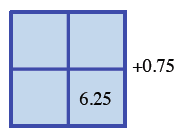### Home > CCA2 > Chapter 2 > Lesson 2.2.5 > Problem2-166

2-166.

Use the technique of completing the square to express $y = x^2 − 5x + 7$ in graphing form and state the vertex.

Write the expressions in their correct locations.

How many ones do you need to complete the square?
How many do you have?

$x$  $-2.5x$  $x$  $-2.5$  $-2.5x$  $x^2$